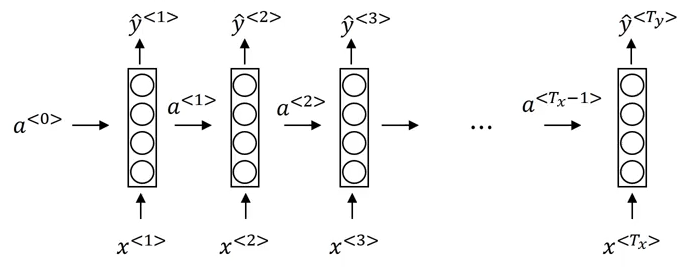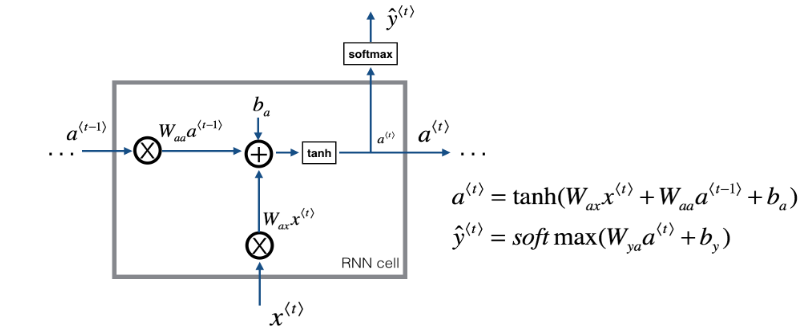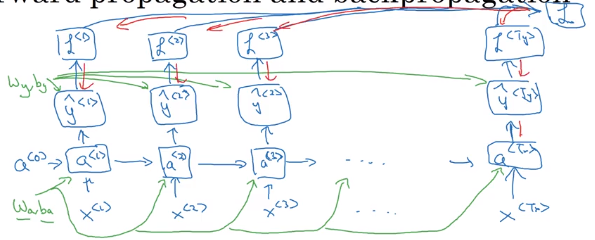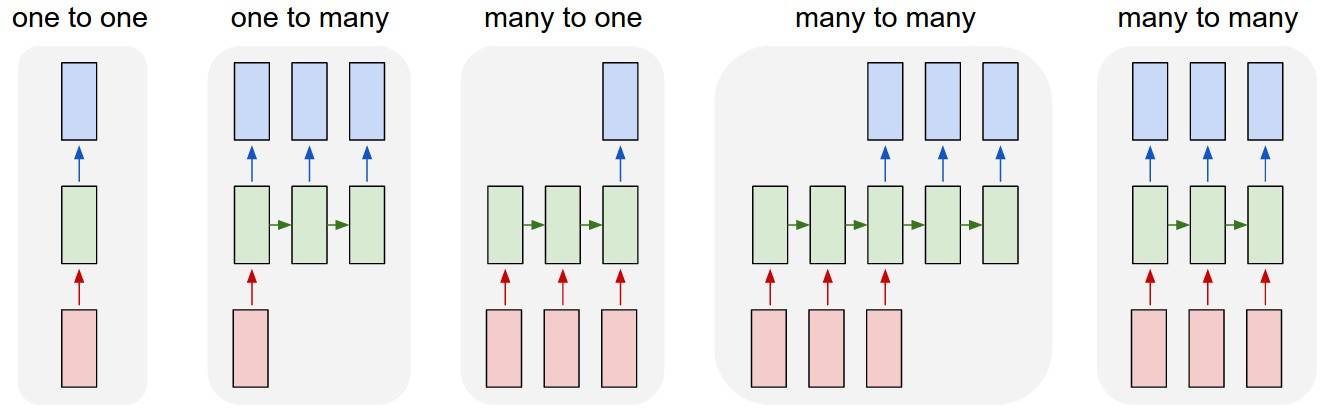# Recurrent Neural Network Basics

Recurrent Neural Networks are designed to learn information from sequential data.

We start with datasets of x time steps in a row, for example:

• x words in a sentence
• x sequential stock ticks
• x days of weather in a row

Thus, we say that there are T_x elements in a given point of data.

In the most basic case, we have some handoff of information, a_i from layer i-1 to i. This gets run through a cell at each step and outputs:

• y_i the prediction at the ith step
• a_i the carry-forward information to the same cell

## Visually

Each cell calculates given information of both, this element as well as the output of the last element

from IPython.display import Image
Image('images/base_rnn.png')Specifically at the cell level, this happens through pairs of Weight matricies and Bias terms.

• W_aa, b_a: to this activation from the last activation
• W_ax, b_x: to this activation from the input data
• W_ya, b_y: (not pictured): to this output from this activation
Image('images/rnn_cell.PNG')The values for W_aa, W_ay, and W_ax are literally the same object, regardless which element, t, you’re looking at.

## Cost Function

The output at each layer has its own cost function that looks like our typical logistic/softmax cost

$\mathcal{L}^{\langle t \rangle}(\hat{y}^{\langle t \rangle}, y^{\langle t \rangle}) = -y^{\langle t \rangle} log \hat{y}^{\langle t \rangle} -(1-y^{\langle t \rangle}) log (1-\hat{y}^{\langle t \rangle})$

The key difference here, however, is that there’s an over-arching cost L that applies across each layer

$\mathcal{L}(\hat{y}, y) = \sum_{t=1}^{T_x} \mathcal{L}^{\langle t \rangle}(\hat{y}^{\langle t \rangle}, y^{\langle t \rangle})$

Image('images/rnn_backprop.png')This allows us a great deal of flexibility in how we construct our Recurrent Networks

## Different Architectures

Image('images/rnn_types.png')• one-to-one: Vanilla MLP
• one-to-many: text generation
• many-to-one: gender prediction based on audio clip
• many-to-many(1): language translation
• many-to-many(2): video classification